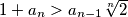### IMO Shortlist 2001 problem A2

Kvaliteta:
Avg: 2,0
Težina:
Avg: 6,0
Let$a_0, a_1, a_2, \ldots$ be an arbitrary infinite sequence of positive numbers. Show that the inequality$1 + a_n > a_{n-1} \sqrt[n]{2}$ holds for infinitely many positive integers$n$.
Izvor: Međunarodna matematička olimpijada, shortlist 2001